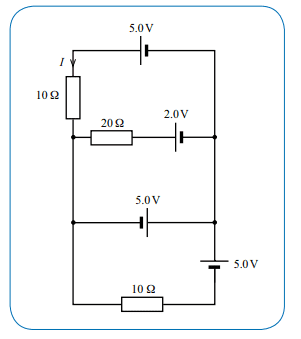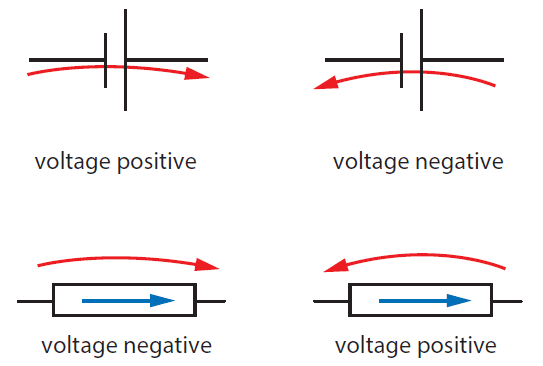# Kirchhoff's Laws

## Homework Statement

Use Kirchhoff's Law to find the current I in the circuit shown below. Choosing the best loop can simplify the problem. (a) Which loop in the circuit should you choose? (b) Calculate the current I## Homework Equations

Kirchhoff's Second Law
∑V = 0

and the junction law
∑I = 0

## The Attempt at a Solution

Firstly, the mark scheme says that the third loop should be used. The reason being that loop is the only loop with the current I going through the resistor. It makes sense to use the loop with I being the only current through the resistor. However, I do not understand how they know that the current through the 10 ohm resistor in the first loop is the same as the current through the 10 ohm resistor in the bottom loop.

Secondly, the mark scheme says I = 1.0 A. I'm assuming that was calculated the following way....

0 = 5 + 5 +10I
I = 1 A.

But that does not make sense to me either. I am using the following rule....When I use that on the bottom loop I get the following -

0 = 5 - 5 - 10I

What am I doing wrong?

Thanks

#### Attachments

haruspex
Homework Helper
Gold Member
the mark scheme says that the third loop should be used.
Not sure what is meant by that. There are six loops (pick any two horizontal connections from four). The best choice I see is the top and third horizontal.
I do not understand how they know that the current through the 10 ohm resistor in the first loop is the same as the current through the 10 ohm resistor in the bottom loop.
It isn't, but we don't care about the current through the bottom resistor.

CWatters
Homework Helper
Gold Member

"neither does current go through BG"

.. is wrong. There is current flowing in BG but you don't need to calculate it.

There are six loops (pick any two horizontal connections from four). The best choice I see is the top and third horizontal

+1

Remember that a voltage source connected between two nodes forces a known voltage to appear between those nodes.